## RD Sharma Solutions for Class 6 Chapter 14 Circles Free Online

Exercise 14.1 page: 14.4
1. Explain the following:
(i) Circle
(iii) Centre
(iv) Diameter
(v) Chord
(vi) Interior of a circle.
Solution:
(i) Circle – A circle is a set of all those points in a plane whose distance from a fixed point remains constant.
(ii) Radius – The radius of a circle is the distance between the all the points of the circle to its centre.
(iii) Centre – The centre of a circle is a fixed point which is at a constant distance from all the points.
(iv) Diameter – A line segment passing through the centre of a circle, and having its end-points on the circle is called a diameter of the circle.
(v) Chord – A line segment with its end-points lying on a circle is called the chord of the circle.
(vi) Interior of a circle – The part of a plane inside the circle consisting of all the points is called the interior of a circle.
2. Take a point on your notebook and draw circle of radii 4 cm, 3 cm and 6.5 cm, each having the same centre O.
Solution:
The figure given below shows circles of 4 cm, 3 cm and 6.5 cm radii having the same centre.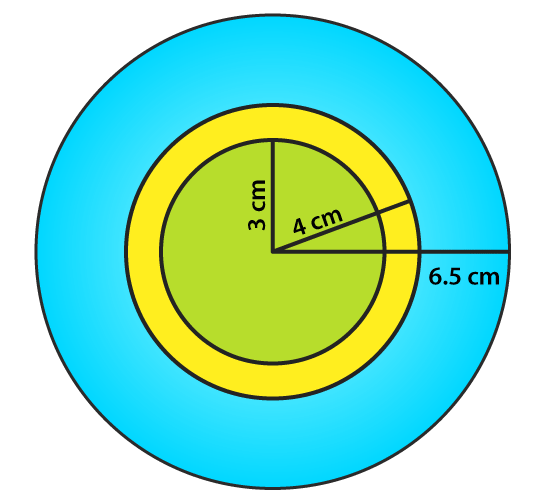3. Draw a circle with centre O and any radius. Draw AC and BD two perpendicular diameters of the circle. Join AB, BC, CD and DA.
Solution:
The figure given below shows a circle with centre O and two perpendicular diameter AC and BC.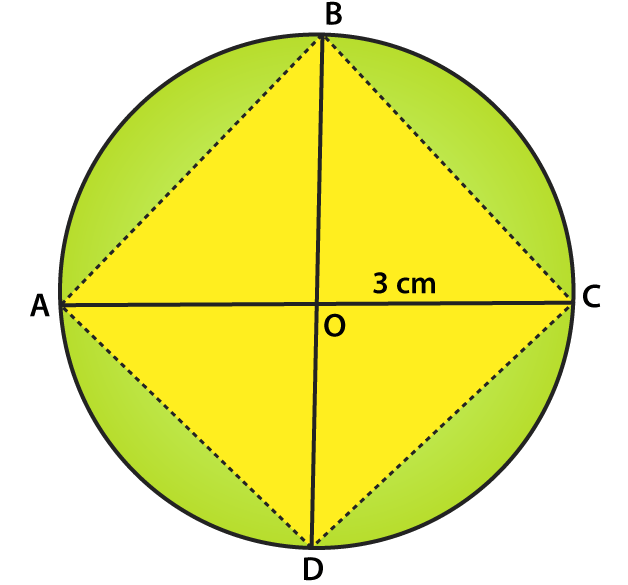4. Draw a circle with centre O and radius 6 cm. Mark points P, Q, R such that
(i) P lies on the circle,
(ii) Q lies in the interior of the circle, and
(iii) R lies in the exterior of the circle.
Rewrite each of the following statements using the correct symbol (=, < or >):
(i) OQ …… 5 cm (ii) OP ……. 5 cm (iii) OR …… 5 cm.
Solution: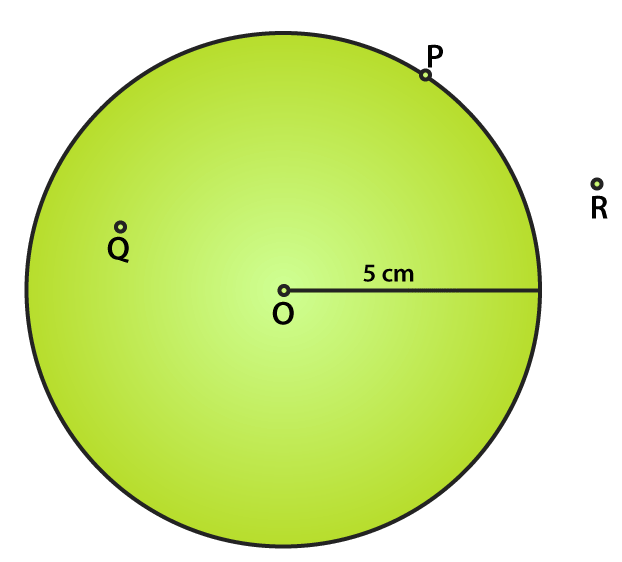The figure given below shows the points P, Q and R such that
(i) P lies on the circle,
(ii) Q lies in the interior of the circle, and
(iii) R lies in the exterior of the circle.
The statements can be written as
(i) OQ < 5 cm
(ii) OP = 5 cm
(iii) OR > 5 cm
5. Take two points A and B on the page of your note book. Draw a circle with centre A which passes through B.
Solution:
The figure given below shows the circle with A as centre and a line which passes through B.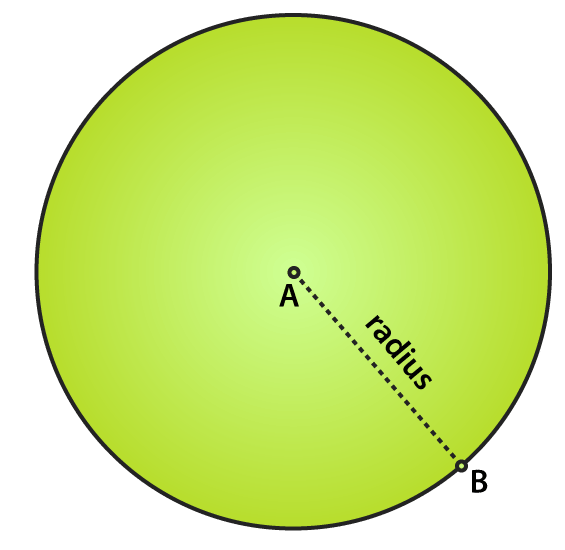6. Draw a semi-circle with centre O and radius 5 cm. Is the diameter that determines the semi-circle, a part of the semi-circle?
Solution:
The figure given below shows a semi-circle with centre O and radius 5 cm.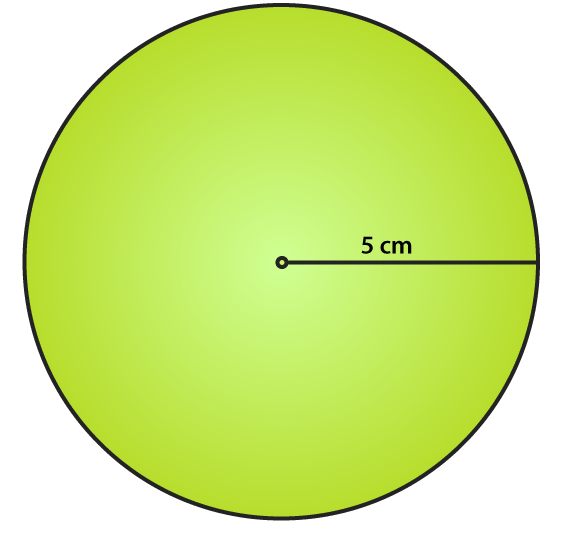We know that a semi-circle is the end point of a diameter which divides the circle into two equal parts.
No, the diameter does not determine the semi-circle and it is the end points of the diameter which finds the semi-circle or a part of the semi-circle.
7. The diameter of a circle is 14 cm, find its radius.
Solution:
It is given that
Diameter of a circle = 14 cm
We know that
Radius of a circle = Diameter / 2
By substituting the values
Radius of a circle = 14/2 = 7 cm.
8. Given a circle with centre O and radius 2.5 cm, what is the length of the longest chord of the circle.
Solution:
We know that the diameter of a circle is its longest chord which is twice its radius.
So the length of the longest chord of the circle = 2 (2.5) = 5 cm.
9. Fill in the blanks:
(i) The diameter of a circle is ……. times its radius.
(ii) The diameter of a circle is the ……. chord of the circle.
(iii) The diameter of a circle pass through ……
(iv) A chord of a circle is a line segment with its end points on the ……
(v) If we join any two points on a circle by a line segment, we obtain …… of the circle.
(vi) A radius of a circle is a line segment with one end at ……. and the other end at …..
(vii) All radii of a circle are ……
(viii) The diameters of a circle are ……
(ix) The total number of diameters of a circle is …..
(x) Every point on a circle is ……. from its centre.
(xi) A chord of a circle contains exactly …… points of the circle.
(xii) A diameter is the longest …….
(xiii) Concentric circles are circles having ……
Solution:
(i) The diameter of a circle is two times its radius.
(ii) The diameter of a circle is the longest chord of the circle.
(iii) The diameter of a circle pass through its centre.
(iv) A chord of a circle is a line segment with its end points on the circle.
(v) If we join any two points on a circle by a line segment, we obtain chord of the circle.
(vi) A radius of a circle is a line segment with one end at centre and the other end at circle.
(vii) All radii of a circle are equal.
(viii) The diameters of a circle are concurrent.
(ix) The total number of diameters of a circle is infinite.
(x) Every point on a circle is equidistant from its centre.
(xi) A chord of a circle contains exactly two points of the circle.
(xii) A diameter is the longest chord.
(xiii) Concentric circles are circles having same centre.
10. In each of the following, state if the statement is true (T) or false (F):
(i) Every circle has a centre.
(ii) The centre of a circle is a point of the circle.
(iii) Any two radii of a circle make up a diameter.
(iv) Every chord of a circle is parallel to some diameter of the circle.
(v) A circle is symmetric about each of its diameters.
(vi) The diameter is twice the radius.
(vii) A radius is a chord of the circle.
(viii) Concentric circles have the same radii.
(ix) The nearer a chord to the centre of a circle, the longer is its length.
Solution:
(i) True.
(ii) False.
(iii) False.
(iv) False.
(v) True.
(vi) True.
(vii) False.
(viii) False.
(ix) True.
Objective Type Questions page: 14.5
Mark the correct alternative in each of the following:
1. A circle of radius r cm has diameter of length
(a) r cm
(b) 2r cm
(c) 4r cm
(d) r/2 cm
Solution:
The option (b) is the correct answer.
A circle of radius r cm has diameter of length 2r cm.
2. A chord of a circle passing through its centre is equal to its
(b) diameter
(c) circumference
(d) none of these
Solution:
The option (b) is the correct answer.
A chord of a circle passing through its centre is equal to its diameter.
3. The total number of diameters of a circle is
(a) 1
(b) 2
(c) 4
(d) uncountable number
Solution:
The option (d) is the correct answer.
The total number of diameters of a circle is uncountable number.
4. By joining any two points on a circle, we obtain its
(b) diameter
(c) chord
(d) circumference
Solution:
The option (c) is the correct answer.
By joining any two points on a circle, we obtain its chord.
5. The longest chord of a circle is equal to its
(b) diameter
(c) circumference
(d) perimeter
Solution:
The option (b) is the correct answer.
The longest chord of a circle is equal to its diameter.
6. How many circles can be drawn to pass through two given points?
(a) 1
(b) 2
(c) 0
(d) As many as possible
Solution:
The option (d) is the correct answer.
Many circles can be drawn to pass through two given points.
7. How many circles can be drawn to pass through three non-collinear points?
(a) 1
(b) 2
(c) 0
(d) As many as possible
Solution:
The option (a) is the correct answer.
The number of circles which can be drawn that pass through three non-collinear points is 1.
Courtesy : CBSE